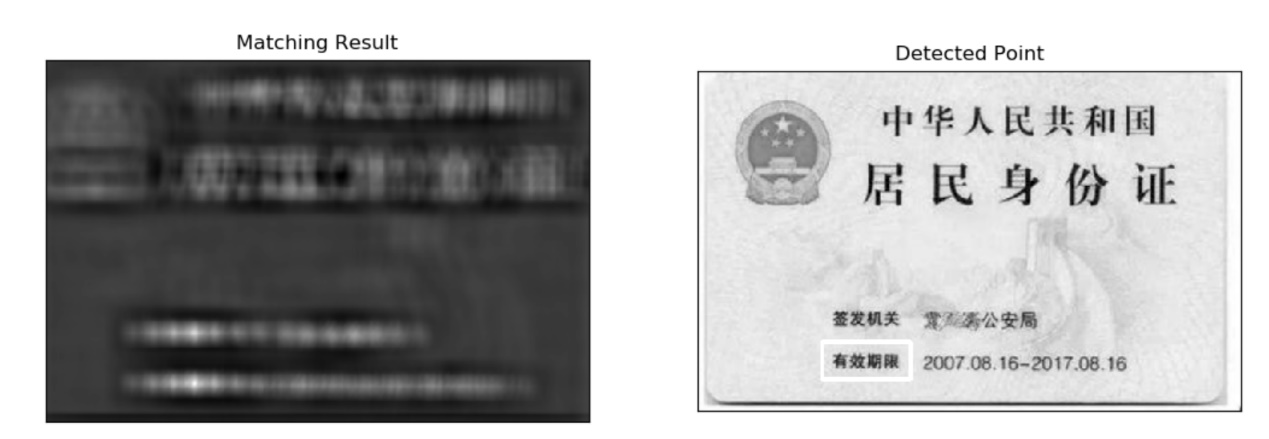# 在OpenCV里实现模板匹配

#python 3.7.4,opencv4.1
#蔡军生 https://blog.csdn.net/caimouse/article/details/51749579
#
import numpy as np
import cv2
from matplotlib import pyplot as plt

img2 = img.copy()
w, h = template.shape[::-1]

#6种比较方法
methods = ['cv2.TM_CCOEFF', 'cv2.TM_CCOEFF_NORMED', 'cv2.TM_CCORR',
'cv2.TM_CCORR_NORMED', 'cv2.TM_SQDIFF', 'cv2.TM_SQDIFF_NORMED']

for meth in methods:
img = img2.copy()
method = eval(meth)#这里把字符串表达式求值，返回宏表达的数值

#匹配比较
res = cv2.matchTemplate(img,template,method)
min_val, max_val, min_loc, max_loc = cv2.minMaxLoc(res)

#TM_SQDIFF 或 TM_SQDIFF_NORMED取最小值有效
if method in [cv2.TM_SQDIFF, cv2.TM_SQDIFF_NORMED]:
top_left = min_loc
else:
top_left = max_loc
bottom_right = (top_left + w, top_left + h)

cv2.rectangle(img,top_left, bottom_right, 255, 3)#画白色矩形作为标记

plt.subplot(121),plt.imshow(res,cmap = 'gray')
plt.title('Matching Result'), plt.xticks([]), plt.yticks([])
plt.subplot(122),plt.imshow(img,cmap = 'gray')
plt.title('Detected Point'), plt.xticks([]), plt.yticks([])
plt.suptitle(meth)

plt.show()res = cv.matchTemplate(img_gray,template,cv.TM_CCOEFF_NORMED)

threshold = 0.8

loc = np.where( res >= threshold)

for pt in zip(*loc[::-1]):

cv.rectangle(img_rgb, pt, (pt + w, pt + h), (0,0,255), 2)

https://blog.csdn.net/caimouse/article/details/51749579

0 个人打赏©️2019 CSDN 皮肤主题: 大白 设计师: CSDN官方博客